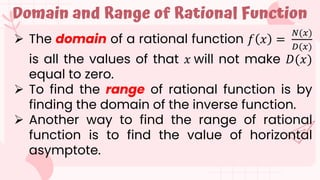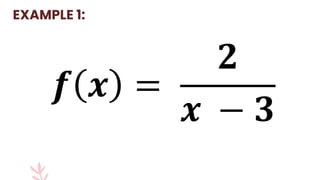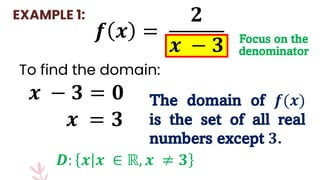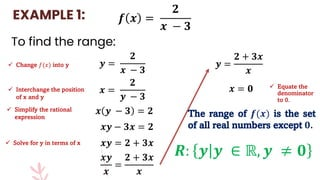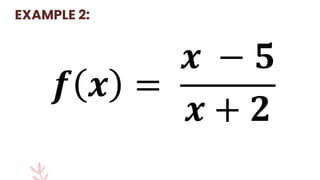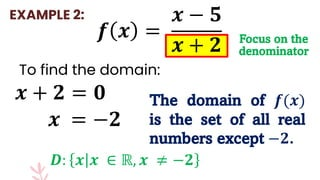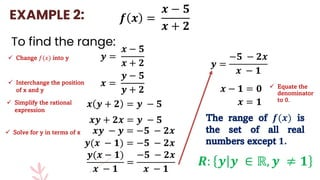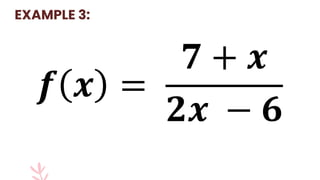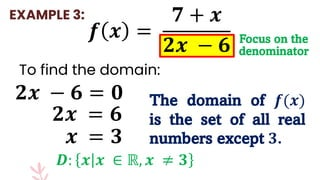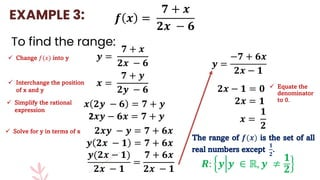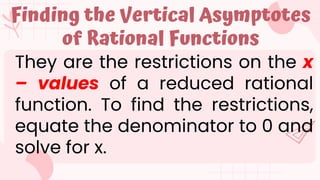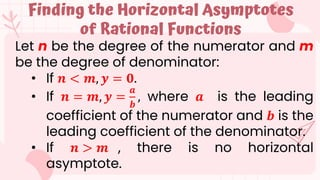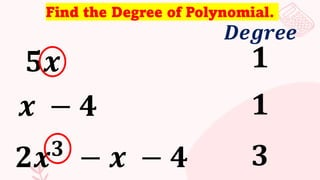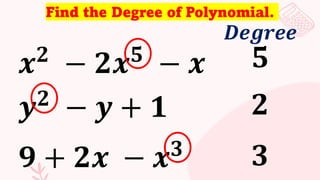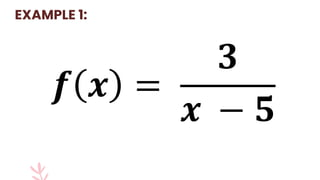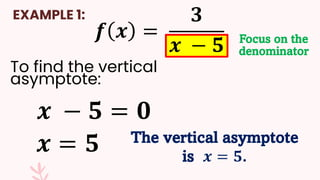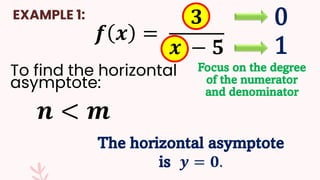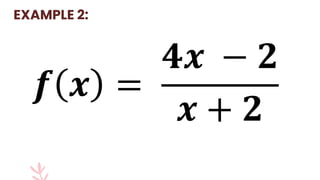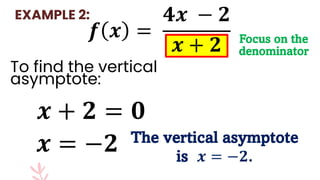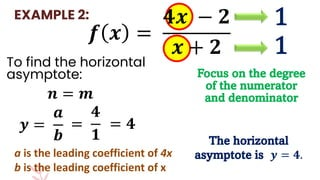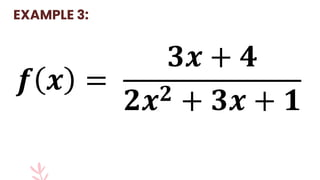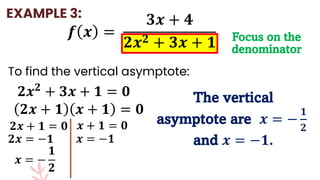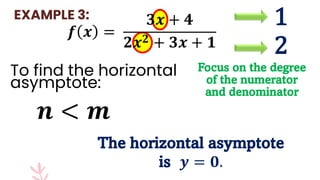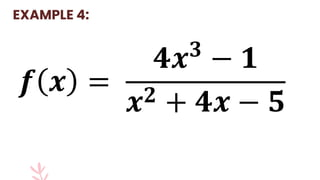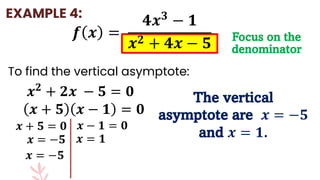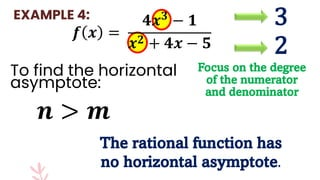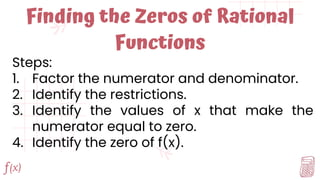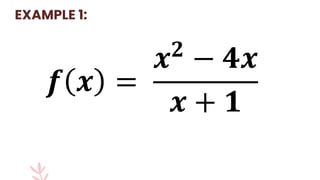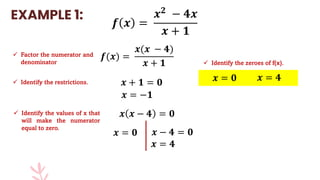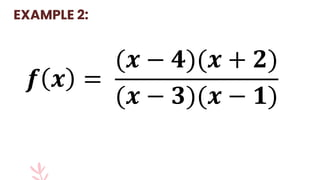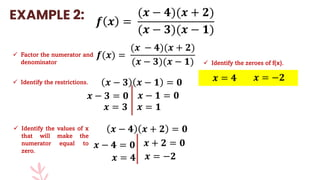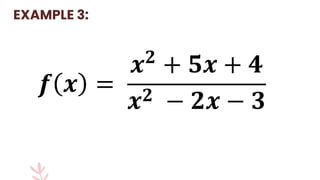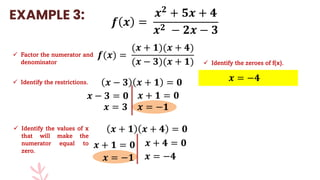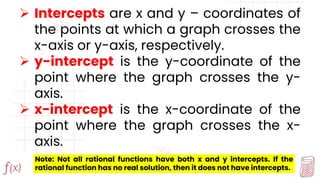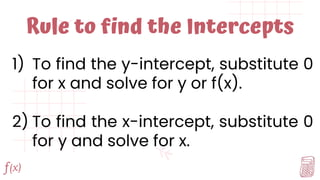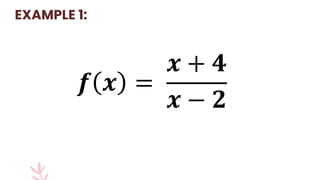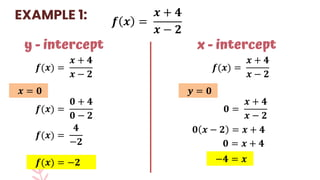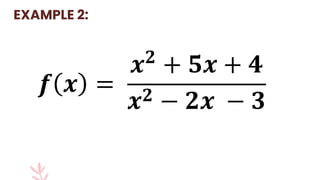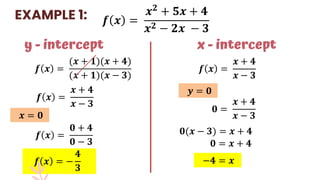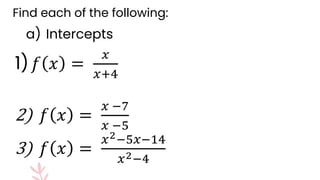1 de 51

### Domain-Range-Intercepts-Zeros-and-Asymptotes-of-Rational-Function.pptx

1. Domain, Range, Zeros, Intercepts and Asymptotes of Rational Function
2. Objectives: find the domain, range, zeroes and intercepts of rational functions determine the vertical and horizontal asymptotes of rational function.
3.  The domain of a rational function 𝑓 𝑥 = 𝑁(𝑥) 𝐷(𝑥) is all the values of that 𝑥 will not make 𝐷(𝑥) equal to zero.  To find the range of rational function is by finding the domain of the inverse function.  Another way to find the range of rational function is to find the value of horizontal asymptote. Domain and Range of Rational Function
4. 𝒇 𝒙 = 𝟐 𝒙 − 𝟑 EXAMPLE 1:
5. 𝒇 𝒙 = 𝟐 𝒙 − 𝟑 𝒙 − 𝟑 = 𝟎 Focus on the denominator The domain of 𝒇(𝒙) is the set of all real numbers except 𝟑. EXAMPLE 1: 𝒙 = 𝟑 To find the domain: 𝑫: 𝒙 𝒙 ∈ ℝ, 𝒙 ≠ 𝟑
6. 𝒇 𝒙 = 𝟐 𝒙 − 𝟑  Change 𝑓(𝑥) into y EXAMPLE 1: To find the range: 𝒚 = 𝟐 𝒙 − 𝟑  Interchange the position of x and y 𝒙 = 𝟐 𝒚 − 𝟑  Simplify the rational expression 𝒙 𝒚 − 𝟑 = 𝟐 𝒙𝒚 − 𝟑𝒙 = 𝟐  Solve for y in terms of x 𝒙𝒚 = 𝟐 + 𝟑𝒙 𝒙𝒚 𝒙 = 𝟐 + 𝟑𝒙 𝒙 𝒚 = 𝟐 + 𝟑𝒙 𝒙  Equate the denominator to 0. 𝒙 = 𝟎 The range of 𝒇(𝒙) is the set of all real numbers except 𝟎. 𝑹: 𝒚 𝒚 ∈ ℝ, 𝒚 ≠ 𝟎
7. 𝒇 𝒙 = 𝒙 − 𝟓 𝒙 + 𝟐 EXAMPLE 2:
8. 𝒇 𝒙 = 𝒙 − 𝟓 𝒙 + 𝟐 𝒙 + 𝟐 = 𝟎 Focus on the denominator The domain of 𝒇(𝒙) is the set of all real numbers except −𝟐. EXAMPLE 2: 𝒙 = −𝟐 To find the domain: 𝑫: 𝒙 𝒙 ∈ ℝ, 𝒙 ≠ −𝟐
9. 𝒇 𝒙 = 𝒙 − 𝟓 𝒙 + 𝟐  Change 𝑓(𝑥) into y EXAMPLE 2: To find the range: 𝒚 = 𝒙 − 𝟓 𝒙 + 𝟐  Interchange the position of x and y 𝒙 = 𝒚 − 𝟓 𝒚 + 𝟐  Simplify the rational expression 𝒙 𝒚 + 𝟐 = 𝒚 − 𝟓 𝒙𝒚 + 𝟐𝒙 = 𝒚 − 𝟓  Solve for y in terms of x 𝒙𝒚 − 𝒚 = −𝟓 − 𝟐𝒙 𝒚(𝒙 − 𝟏) 𝒙 − 𝟏 = −𝟓 − 𝟐𝒙 𝒙 − 𝟏 𝒚 = −𝟓 − 𝟐𝒙 𝒙 − 𝟏  Equate the denominator to 0. 𝒙 − 𝟏 = 𝟎 The range of 𝒇(𝒙) is the set of all real numbers except 𝟏. 𝑹: 𝒚 𝒚 ∈ ℝ, 𝒚 ≠ 𝟏 𝒚(𝒙 − 𝟏) = −𝟓 − 𝟐𝒙 𝒙 = 𝟏
10. 𝒇 𝒙 = 𝟕 + 𝒙 𝟐𝒙 − 𝟔 EXAMPLE 3:
11. 𝒇 𝒙 = 𝟕 + 𝒙 𝟐𝒙 − 𝟔 𝟐𝒙 − 𝟔 = 𝟎 Focus on the denominator The domain of 𝒇(𝒙) is the set of all real numbers except 𝟑. EXAMPLE 3: 𝟐𝒙 = 𝟔 To find the domain: 𝑫: 𝒙 𝒙 ∈ ℝ, 𝒙 ≠ 𝟑 𝒙 = 𝟑
12. 𝒇 𝒙 = 𝟕 + 𝒙 𝟐𝒙 − 𝟔  Change 𝑓(𝑥) into y EXAMPLE 3: To find the range: 𝒚 = 𝟕 + 𝒙 𝟐𝒙 − 𝟔  Interchange the position of x and y 𝒙 = 𝟕 + 𝒚 𝟐𝒚 − 𝟔  Simplify the rational expression 𝒙 𝟐𝒚 − 𝟔 = 𝟕 + 𝒚 2𝒙𝒚 − 𝟔𝒙 = 𝟕 + 𝒚  Solve for y in terms of x 𝟐𝒙𝒚 − 𝒚 = 𝟕 + 𝟔𝒙 𝒚(𝟐𝒙 − 𝟏) 𝟐𝒙 − 𝟏 = 𝟕 + 𝟔𝒙 𝟐𝒙 − 𝟏 𝒚 = −𝟕 + 𝟔𝒙 𝟐𝒙 − 𝟏  Equate the denominator to 0. 𝟐𝒙 − 𝟏 = 𝟎 The range of 𝒇(𝒙) is the set of all real numbers except 𝟏 𝟐 . 𝑹: 𝒚 𝒚 ∈ ℝ, 𝒚 ≠ 𝟏 𝟐 𝒚 𝟐𝒙 − 𝟏 = 𝟕 + 𝟔𝒙 𝟐𝒙 = 𝟏 𝒙 = 𝟏 𝟐
13. Vertical and Horizontal Asymptotes of Rational Functions
14. They are the restrictions on the x – values of a reduced rational function. To find the restrictions, equate the denominator to 0 and solve for x. Finding the Vertical Asymptotes of Rational Functions
15. Let n be the degree of the numerator and m be the degree of denominator: • If 𝒏 < 𝒎, 𝒚 = 𝟎. • If 𝒏 = 𝒎, 𝒚 = 𝒂 𝒃 , where 𝒂 is the leading coefficient of the numerator and 𝒃 is the leading coefficient of the denominator. • If 𝒏 > 𝒎 , there is no horizontal asymptote. Finding the Horizontal Asymptotes of Rational Functions
16. Find the Degree of Polynomial. 𝟓𝒙 𝟏 𝑫𝒆𝒈𝒓𝒆𝒆 𝒙 − 𝟒 𝟏 𝟐𝒙𝟑 − 𝒙 − 𝟒 𝟑
17. Find the Degree of Polynomial. 𝒙𝟐 − 𝟐𝒙𝟓 − 𝒙 𝟓 𝑫𝒆𝒈𝒓𝒆𝒆 𝒚𝟐 − 𝒚 + 𝟏 𝟐 𝟗 + 𝟐𝒙 − 𝒙𝟑 𝟑
18. EXAMPLE 1: 𝒇 𝒙 = 𝟑 𝒙 − 𝟓
19. 𝒇 𝒙 = 𝟑 𝒙 − 𝟓 To find the vertical asymptote: 𝒙 − 𝟓 = 𝟎 𝒙 = 𝟓 Focus on the denominator The vertical asymptote is 𝒙 = 𝟓. EXAMPLE 1:
20. 𝒇 𝒙 = 𝟑 𝒙 − 𝟓 To find the horizontal asymptote: 𝒏 < 𝒎 Focus on the degree of the numerator and denominator The horizontal asymptote is 𝒚 = 𝟎. 0 1 EXAMPLE 1:
21. 𝒇 𝒙 = 𝟒𝒙 − 𝟐 𝒙 + 𝟐 EXAMPLE 2:
22. 𝒇 𝒙 = 𝟒𝒙 − 𝟐 𝒙 + 𝟐 To find the vertical asymptote: 𝒙 + 𝟐 = 𝟎 𝒙 = −𝟐 Focus on the denominator The vertical asymptote is 𝒙 = −𝟐. EXAMPLE 2:
23. 𝒇 𝒙 = 𝟒𝒙 − 𝟐 𝒙 + 𝟐 To find the horizontal asymptote: 𝒏 = 𝒎 Focus on the degree of the numerator and denominator The horizontal asymptote is 𝒚 = 𝟒. 1 1 EXAMPLE 2: 𝒚 = 𝒂 𝒃 = 𝟒 𝟏 = 𝟒 a is the leading coefficient of 4x b is the leading coefficient of x
24. 𝒇 𝒙 = 𝟑𝒙 + 𝟒 𝟐𝒙𝟐 + 𝟑𝒙 + 𝟏 EXAMPLE 3:
25. 𝒇 𝒙 = 𝟑𝒙 + 𝟒 𝟐𝒙𝟐 + 𝟑𝒙 + 𝟏 To find the vertical asymptote: 𝟐𝒙𝟐 + 𝟑𝒙 + 𝟏 = 𝟎 Focus on the denominator The vertical asymptote are 𝒙 = − 𝟏 𝟐 and 𝒙 = −𝟏. EXAMPLE 3: 𝟐𝒙 + 𝟏 𝒙 + 𝟏 = 𝟎 𝟐𝒙 + 𝟏 = 𝟎 𝒙 + 𝟏 = 𝟎 𝟐𝒙 = −𝟏 𝒙 = − 𝟏 𝟐 𝒙 = −𝟏
26. 1 2 EXAMPLE 3: 𝒇 𝒙 = 𝟑𝒙 + 𝟒 𝟐𝒙𝟐 + 𝟑𝒙 + 𝟏 To find the horizontal asymptote: 𝒏 < 𝒎 Focus on the degree of the numerator and denominator The horizontal asymptote is 𝒚 = 𝟎.
27. 𝒇 𝒙 = 𝟒𝒙𝟑 − 𝟏 𝒙𝟐 + 𝟒𝒙 − 𝟓 EXAMPLE 4:
28. 𝒇 𝒙 = 𝟒𝒙𝟑 − 𝟏 𝒙𝟐 + 𝟒𝒙 − 𝟓 To find the vertical asymptote: 𝒙𝟐 + 𝟐𝒙 − 𝟓 = 𝟎 Focus on the denominator The vertical asymptote are 𝒙 = −𝟓 and 𝒙 = 𝟏. EXAMPLE 4: 𝒙 + 𝟓 𝒙 − 𝟏 = 𝟎 𝒙 + 𝟓 = 𝟎 𝒙 − 𝟏 = 𝟎 𝒙 = −𝟓 𝒙 = 𝟏 𝒙 = −𝟓
29. 3 2 EXAMPLE 4: To find the horizontal asymptote: 𝒏 > 𝒎 Focus on the degree of the numerator and denominator The rational function has no horizontal asymptote. 𝒇 𝒙 = 𝟒𝒙𝟑 − 𝟏 𝒙𝟐 + 𝟒𝒙 − 𝟓
30. Zeros of Rational Function
31. Finding the Zeros of Rational Functions Steps: 1. Factor the numerator and denominator. 2. Identify the restrictions. 3. Identify the values of x that make the numerator equal to zero. 4. Identify the zero of f(x).
32. 𝒇 𝒙 = 𝒙𝟐 − 𝟒𝒙 𝒙 + 𝟏 EXAMPLE 1:
33. 𝒇 𝒙 = 𝒙𝟐 − 𝟒𝒙 𝒙 + 𝟏  Factor the numerator and denominator EXAMPLE 1: 𝒇(𝒙) = 𝒙(𝒙 − 𝟒) 𝒙 + 𝟏  Identify the restrictions. 𝒙 + 𝟏 = 𝟎 𝒙 = −𝟏  Identify the values of x that will make the numerator equal to zero. 𝒙 𝒙 − 𝟒 = 𝟎 𝒙 = 𝟎 𝒙 − 𝟒 = 𝟎 𝒙 = 𝟒  Identify the zeroes of f(x). 𝒙 = 𝟎 𝒙 = 𝟒
34. 𝒇 𝒙 = (𝒙 − 𝟒)(𝒙 + 𝟐) (𝒙 − 𝟑)(𝒙 − 𝟏) EXAMPLE 2:
35.  Factor the numerator and denominator EXAMPLE 2: 𝒇(𝒙) = (𝒙 − 𝟒)(𝒙 + 𝟐) (𝒙 − 𝟑)(𝒙 − 𝟏)  Identify the restrictions. 𝒙 − 𝟑 𝒙 − 𝟏 = 𝟎 𝒙 − 𝟑 = 𝟎  Identify the values of x that will make the numerator equal to zero. 𝒙 − 𝟒 𝒙 + 𝟐 = 𝟎 𝒙 − 𝟒 = 𝟎 𝒙 + 𝟐 = 𝟎 𝒙 = −𝟐  Identify the zeroes of f(x). 𝒙 = 𝟒 𝒙 = −𝟐 𝒇 𝒙 = (𝒙 − 𝟒)(𝒙 + 𝟐) (𝒙 − 𝟑)(𝒙 − 𝟏) 𝒙 = 𝟑 𝒙 − 𝟏 = 𝟎 𝒙 = 𝟏 𝒙 = 𝟒
36. 𝒇 𝒙 = 𝒙𝟐 + 𝟓𝒙 + 𝟒 𝒙𝟐 − 𝟐𝒙 − 𝟑 EXAMPLE 3:
37.  Factor the numerator and denominator EXAMPLE 3: 𝒇(𝒙) = (𝒙 + 𝟏)(𝒙 + 𝟒) (𝒙 − 𝟑)(𝒙 + 𝟏)  Identify the restrictions. 𝒙 − 𝟑 𝒙 + 𝟏 = 𝟎 𝒙 − 𝟑 = 𝟎  Identify the values of x that will make the numerator equal to zero. 𝒙 + 𝟏 𝒙 + 𝟒 = 𝟎 𝒙 + 𝟏 = 𝟎 𝒙 + 𝟒 = 𝟎 𝒙 = −𝟒  Identify the zeroes of f(x). 𝒙 = −𝟒 𝒙 = 𝟑 𝒙 + 𝟏 = 𝟎 𝒙 = −𝟏 𝒙 = −𝟏 𝒇 𝒙 = 𝒙𝟐 + 𝟓𝒙 + 𝟒 𝒙𝟐 − 𝟐𝒙 − 𝟑
38. Intercepts of Rational Functions
39.  Intercepts are x and y – coordinates of the points at which a graph crosses the x-axis or y-axis, respectively.  y-intercept is the y-coordinate of the point where the graph crosses the y- axis.  x-intercept is the x-coordinate of the point where the graph crosses the x- axis. Note: Not all rational functions have both x and y intercepts. If the rational function has no real solution, then it does not have intercepts.
40. Rule to find the Intercepts 1) To find the y-intercept, substitute 0 for x and solve for y or f(x). 2) To find the x-intercept, substitute 0 for y and solve for x.
41. 𝒇 𝒙 = 𝒙 + 𝟒 𝒙 − 𝟐 EXAMPLE 1:
42. 𝒇 𝒙 = 𝒙 + 𝟒 𝒙 − 𝟐 EXAMPLE 1: y - intercept 𝒇(𝒙) = 𝒙 + 𝟒 𝒙 − 𝟐 𝒙 = 𝟎 𝒇(𝒙) = 𝟎 + 𝟒 𝟎 − 𝟐 𝒇(𝒙) = 𝟒 −𝟐 𝒇(𝒙) = −𝟐 x - intercept 𝒇(𝒙) = 𝒙 + 𝟒 𝒙 − 𝟐 𝒚 = 𝟎 𝟎 = 𝒙 + 𝟒 𝒙 − 𝟐 𝟎 𝒙 − 𝟐 = 𝒙 + 𝟒 𝟎 = 𝒙 + 𝟒 −𝟒 = 𝒙
43. 𝒇 𝒙 = 𝒙𝟐 + 𝟓𝒙 + 𝟒 𝒙𝟐 − 𝟐𝒙 − 𝟑 EXAMPLE 2:
44. EXAMPLE 1: y - intercept 𝒙 = 𝟎 𝒇 𝒙 = 𝟎 + 𝟒 𝟎 − 𝟑 x - intercept 𝒚 = 𝟎 𝟎 = 𝒙 + 𝟒 −𝟒 = 𝒙 𝒇 𝒙 = 𝒙𝟐 + 𝟓𝒙 + 𝟒 𝒙𝟐 − 𝟐𝒙 − 𝟑 𝒇 𝒙 = (𝒙 + 𝟏)(𝒙 + 𝟒) (𝒙 + 𝟏)(𝒙 − 𝟑) 𝒇 𝒙 = 𝒙 + 𝟒 𝒙 − 𝟑 𝒇 𝒙 = − 𝟒 𝟑 𝒇 𝒙 = 𝒙 + 𝟒 𝒙 − 𝟑 𝟎 = 𝒙 + 𝟒 𝒙 − 𝟑 𝟎(𝒙 − 𝟑) = 𝒙 + 𝟒
45. Find each of the following: a) Intercepts 1)𝑓 𝑥 = 𝑥 𝑥+4 2) 𝑓 𝑥 = 𝑥 −7 𝑥 −5 3) 𝑓 𝑥 = 𝑥2−5𝑥−14 𝑥2−4